1.0   Coplanar lines that don’t intersect

Euclid defined parallel as:

“ Parallel straight lines are straight lines which lie in the same plane and, if
produced indefinitely in diametrical directions, do not meet in either
direction.” (Bk. 1, Def. 23) 

His definition has barely changed in over 2000 years. Euclid’s disciples continue to define parallel lines as:

“ parallel lines: Straight lines in the same plane that do not meet no matter
how far they are extended.”   

“ Parallel lines are coplanar lines that never intersect.”  

I have essentially four objections to these notions. I analyze them under the headings: proof, opinion, infinity,
and segment.

Proof

Whether two lines meet at infinity or not is an issue of proof and not of definitions. Indeed, for centuries
mathematicians have tried to 'prove' Euclid's 5th Postulate, which is simply a rewording of his alleged 23rd
definition:

“ That, if a straight line falling on two straight lines makes the interior angles
on the same side less than two right angles, the two straight lines, if
produced indefinitely, meet on that side on which are the angles less than
the two right angles.” (Bk. I, 5th Post.)   

Euclid’s ‘definition’ requires the ET to wait 'til kingdom come' while the mathematician extends his straight lines
indefinitely. Euclid's parallel lines do not constitute a pattern that we can synthesize in a static photograph. The
Euclidean parallel is a movie of two lines that are growing from frame to frame. Euclid's motion picture is still in
production, and we can take no decision until it's finished. In fact, it is guaranteed never to make it to the big
screen.

Opinion

According to another version, whether Euclid’s two lines are parallel ultimately depends on the perception of the
observer. Some relativists contend that railroad tracks that remain ideally parallel throughout their entire extent
and that never touch each other are NOT parallel if from the observer’s perspective the tracks 'appear' to meet in
the horizon!

“ You are wrong to claim that ‘Parallel Lines are precisely the same distance
apart, at any point on the lines,’ because this contradicts our everyday
experience. Just recollect the standard depiction of a pair of railroad tracks
- the farther you look, the closer they become.” 

This is a good example of the inadequacy of making definitions contingent upon proofs and observers. How
far should we extend two 'infinite' lines before we certify that they are parallel? What if a drunk mathematician
decides that two lines are not parallel when in fact they are and vice versa? What if a blind man says they are
parallel? There should be no provision for observers in an objective definition.

Infinity

In Physics, there are no objects that are infinite because this leads to a contradiction with the word object.
An object is by definition finite. There is no such thing as an infinite table as there is no such thing as an
infinite line. All objects must have a background that encloses them all around.

Nevertheless, for the purposes of Science, the word infinite is an adjective and may only be used in the context
of physical objects. In Science, words such as love and energy cannot be infinite, and neither can an itinerary.
If the topic of discussion embodies motion, Science absolutely demands the use of adverbs. Love, energy, and
itineraries may at best be incessant, perpetual, or constant.

The problem with Euclid’s indefinite ‘infinity’ is that he and his disciples have converted this adjective into a
noun. The table is no longer infinite. A part of the table is now located at infinity:

“A point lying on the line at infinity is a point at infinity.”  

[And where is that, may I ask?]

The mathematician is staring at the distant horizon and saying that he cannot see the end of the table.

This vague reply raises several questions. How far should the mathematician travel until he is satisfied that the
table ends? That the table ends is beyond question. A physical object is that which is contoured all around by
an unspecified medium. Otherwise it is not a physical object, but rather a concept. Therefore, the mathematician
should simply walk until he sees the end of the table. Until he sees the end of the table, he cannot yet talk about
a table, and much less about infinity. A line and a plane are no different. If a line and a plane want to be physical
objects – i.e., geometric figures – they cannot be infinite. And if the end of the line is located at infinity, we should
simply go to this popular mathematical place and certify that the line ends before calling it a line.

The problem, say the mathematicians, is that when they attempt to reach the horizon, a new horizon stretches
indefinitely before them. Infinity is a never-ending, surrealistic movie of an alleged physical object that grows
faster than we can approach it. What does this irrational concept have to do with the real world? What does it
have to do with Geometry, a discipline that studies shapes?

Actually, when the mathematicians use the word infinite they are not referring to physical aspects of lines and
planes, but to numbers. They are not saying that the line is infinite or that it has ‘infinite’ points. They are saying
that they can conceivably count an indefinite number of apples. They count points on a line and proclaim that
they see no conceptual end to this counting. They call this infinite line the number line. The points being counted
are not dots, but locations. Sometimes the mathematicians mean the locations of different 'points,' in which case
we are talking about a photograph. Sometimes they mean the successive locations of a single dynamic 'point,'
in which case we are talking about a movie. In this last case, what is 'incessant' is the film. We are incessantly
counting the frames of a never-ending movie.

The mathematicians use the self-serving word infinity to leave their physical interpretations open ended. This
word enables them to sidestep the crucial questions that would challenge the validity of their reasoning. What
happened at the beginning of time or at the Big Bang? Infinity! What is the center of a black hole or what is
located at the center of a black hole? Infinity! Do two parallel lines touch each other? Infinity! We’ve never been
there, so we don’t know, and we can’t prove, so please don’t ask, because it makes no sense to ask, because it
is irrelevant:

“ Nonetheless, your father is wrong to claim that the tracks meet at infinity.
There is no such physical location; and, in any event, no one was close
enough to check although appearances all point to the possibility of the
tracks meeting at ‘infinity.’ ”  

[How much bullshit can a person take?]

[Translation: One lunatic tells the other ‘I see the train tracks meeting in the
horizon. They must touch. I wonder how the train makes it through.’ The
other loon replies ‘You are wrong. The horizon doesn’t exist. I know because
every time I approach it, a new horizon extends before me. But maybe you’re
right. Maybe further down the road the tracks do cross. Who knows? Just in
case, I wouldn’t recommend that you take this train.’]

[ What a bunch of morons the mathematicians of this world are!]

Segment

A line segment is defined as:

“ a portion of a line delimited by two end points”  

“ a part of a straight line between two points on the line. The line itself
extends infinitely in two directions, but the segment is finite.”   

In other words, a line segment is specifically defined as a finite geometric figure.

Assume now that we want to know whether two line segments are parallel. The mathematical definition of
parallel would require us to stretch them to infinity. But this violates the definition of line segment. Pursuant to
the mathematical definition of parallel, we can never tell whether two line segments are parallel unless we
retroactively amend the definition and allow the mathematician to extend them. We must first convert them into
mathematical lines (i.e., infinite) before we can certify this.

But then, if the mathematician is going to extend the line segment and turn it into an infinite line at his
convenience to explain a theory, if he is not going to respect the definition, then why have definitions? The
devil's advocate only wants to know whether the two line segments that stand before us are parallel. With
what authority is the mathematician extending the line segments if line segments are by definition finite?

2.0   Points in common

A better version of Euclid’s parallel lines is ‘lines that do not have a point in common.’

“ In Geometry, the definition of parallel lines is exactly that two lines are parallel
iff they have no common points…in Euclidean Geometry, parallel lines stay
on the ‘same distance from each other’. In other geometries this is not
necessarily so.”  

“ parallel lines meet at infinity and therefore are to be drawn that way… two
parallel lines will meet on a horizon in virtue of their possessing the same
direction.”  

“ P is the point on the line AB such that PA/PB = 1. It can also be thought of as
the point of intersection of two parallel lines.”  

[The point of intersection of two parallel lines? I thought lines are parallel if they
don't intersect? The mathematicians come up with so many exceptions and
amendments to their definitions that they lose all significance. After 3,000 years
of Mathematics, not a single mathematician on Earth can tell you today what
the term parallel means!]

This definition differs from the ‘coplanar lines that don’t intersect’ in that it is static. The ‘don’t intersect’
definition requires us to extend the line to determine whether they intersect. The ‘point in common’ is more
consistent with the definition of the word line. If a line is alleged to be infinite, there is no possibility of
extending it further. We simply accept the hypothesis at face value and verify whether the two lines have a
common point. For this we do not have to watch a movie of an observer desperately seeking a common point.
We can tell by simply looking at the picture whether they have a point in common or not. If the lines have a
common point, some mathematicians tell us that, by definition, the two lines are not parallel.

Again, the problem can be traced to the misconceived definition of the term straight line. We must settle
whether a line is made of dots or locations, whether it can be infinite, whether it is one-dimensional, whether
it is a geometric figure, and whether it is a series of dots or a movie of a single dynamic dot. The line of
Physics is not made of points. It is straight and 'made' of a single piece. The line of Physics is a geometric
figure. The mathematical line is a blend of many irreconcilable concepts, but the most relevant one to the
instant issue is that it is not a geometric figure. The mathematicians are referring to motion. They are
pondering whether your trajectory 'intersected' my itinerary while we were walking around a sphere. They
are not talking about lines. They are talking about geodesics. Therefore, this mathematical definition should
be placed on the back burner until the mathematicians clarify what they are referring to.

However, a more interesting issue with the 'don't intersect' definition is that it allows us to objectively settle
whether two line segments are parallel. I raise the following objections against the 'don't intersect' criterion:

It is a proof and not a definition of parallel

The mathematicians ask us to verify whether there is a point in common. They are saying
that if two line segments extend around the Earth, the ET must track them down until he
finds a common point. What happens if we reach infinity and still the lines are converging,
but still don’t touch? Or should we conclude in the alternative that we are not yet at infinity?

Two line segments may not have point in common and yet not be parallel.

Euclid concocted his definition of parallel for the purposes of plane geometry. However,
since the advent of hyperbolic (Lobachevsky) and elliptic (Riemann) geometry, the essence
of Euclid's definition of parallel'lines that do not meet' – was extended to lines lying
on solids. This created major problems in communication between the subsequent
generations of Mathematicians because every strategic definition of Geometry was now
revised. Henceforth, when someone mentioned the words parallel or perpendicular, everyone
understood something different. It all depended on what particular geometric figure the
lines were sitting on.

So where does the ridiculous relativistic definition leave Euclid's imperfect attempt (from
which it was extrapolated)? In plane (or Euclidean) geometry, parallel and equidistant lines
are synonymous. Irrespective of how far Euclid extends them, it seems reasonable to
conclude that they will not only never meet, but that they will also maintain the same
distance from edge to edge throughout. Not so in hyperbolic and elliptic geometry! The
reason for this is that relativists have changed the rules. Relativists insist that the concept
known as 'straight line' should conform to the physical medium it rests upon. [I believe this
to be the first documented instance in history where man physically bends a concept.]
The way they 'prove' this to novices is by drawing parallel lines on a paper and molding the
paper into a saddle. If the experiment was performed correctly, the two lines should diverge,
which shows that despite not being equidistant they are nevertheless parallel (i.e., they do
not meet).

What relativists have overlooked in their 'empirical' definition is that it relies on the
concept 'straight line'. It turns out that a straight line stands on its own and does not need
a plane or a solid as a backdrop. A straight line has its own definition and cannot be
changed retroactively to suit the prosecutor's theory. The definition of straight line makes
no specific provision for a backdrop. The idiots of Mathematics put in the background by
hand. In fact, if a line required another geometric figure in would not be an object, but a
relation between two of them. Likewise, the various contemporary definitions of the
adjective parallel fail to make provisions for a second geometric figure. Hence, relativists
can define parallel as they wish, but if their definition invokes the term 'straight line', they
cannot fold this definition in their 'saddle' paper-experiment. The definition of straight line
changes neither because it lies on a cube nor on a sphere. A straight line that lies on the
face of a cube remains just as straight as it does when it forms a tangent with the surface
of a sphere. The way we 'prove' this in Physics is by removing the solid on which the
mathematicians drew their lines so as not to distract them (Fig. 1). We discover when we
do this that what the relativist actually did was to modify the definition of the concept
straight line retroactively the moment he bent the physical paper.

The line the mathematicians are talking about, again, is not a stand-alone geometric figure, but an itinerary
around the sphere. Only then does the context become clear. The ‘infinite’ line of Mathematics is dynamic.
It means that a traveler walks incessantly.

But then, does it make sense to say that two 'itineraries' are parallel? Whether they sit on a sphere or not?
Parallel is an adjective applicable to structure. Here, the mathematicians are not talking about structure. They
are talking about motion.  Their alleged line is a movie of an itinerary. So what sense does it make to say that
the frames of a film are parallel to each other? Each of the points with which the mathematicians build their
lines in the first place occupies a single frame of the film. A point is conceptually not an extended object.
Therefore, it makes no sense to invoke the adjective parallel in the context of motion or of footprints left in
the sand.

Nevertheless, whether a Great Circle is regarded as a series of points or an itinerary, it has a beginning
location and an ending one. If our stroll around the world begins at the Eiffel Tower, it must end at the Eiffel
Tower minus one step. If relativists want to go around again, their incessant walk does not make the Great
Circle any longer. But the real issue is that they would be talking about motion and not about a standalone
geometric figure called a curve.

“ In non-Euclidean geometry it is more common to talk about geodesics
than (straight) lines. A geodesic is the path that a particle follows if no
force is applied to it.” In non-Euclidean geometry (spherical or hyperbolic)
the above three definitions are not equivalent: only the second one is
useful in other geometries. In general, equidistant lines are not geodesics
so the equidistant definition cannot be used. In the Euclidean plane, when
two geodesics (straight lines) are intersected with the same angles by a
transversal geodesic (see image), every (non-parallel) geodesic intersects
them with the same angles. In both the hyperbolic and spherical plane, this
is not the case. E.g. geodesics sharing a common perpendicular only do
so at one point (hyperbolic space) or at two (antipodal) points (spherical
space).”  

mathematicians in Germany and Russia started with Euclid's geometry,
but asked: "What if parallel lines _could_ intersect each other? How would
that change things?" And they ended up inventing an entirely new branch
of geometry”  

So if Euclid's definition had flaws, by conserving its essence – intersection – and by further changing the
rules, relativists did not come up with a better mouse trap. It would have been less embarrassing for relativists
to apologize and fix the vase after Euclid dropped it than to try to convince the owner that the scattered pieces
constitute a new type of art.

3.0   The definition of Physics

In Physics, we don't use two lines to define the word parallel. Two lines have unnecessary material that just
distracts us from our quest. The minimum requirements to define the strategic term parallel in Physics are
two edges. Two edges form a pattern, the boundaries of unfinished objects.

Therefore, I will revamp Euclid's erroneous definition and have parallel designate in Physics what most people
understand for parallel in everyday life: equidistant edges. This does not mean that we will measure from edge
to edge to prove whether the definition holds its ground. This would amount to a proof definition. In Physics,
it works in reverse. Two edges are parallel if and only if two edges are equidistant throughout.

parallel: Equidistant straight edges throughout the length of the shortest one,
where the distance is regarded in the direction in which the edge faces.

Distance is a static concept. In Physics, we don't measure distance. In Physics, distance is the separation
between two surfaces. We can also extend this concept to edges because edges necessarily are part of 3-D
geometric figures. There is no such thing as an edge or a plane figure all by itself.

Distance is also an observer-free concept. There is distance between two walls whether anyone is there or
not. Therefore, two edges are equidistant irrespective of observers. In Science, when a prosecutor states
that two objects are parallel, she means that the distance from edge to edge, the gap that separates locations
facing each other, is the same throughout. Now she can use the term 'parallel' consistently in her dissertation.

Note that there is no provision for a backdrop in this definition. The edges belong to the same cross-section
by definition and not because a geometric figure stands in the background. If the edges are not in the same
'plane,' they will necessarily fail to maintain the same distance throughout.

Again, whether this definition is useful to mathematicians is irrelevant. This is what we mean by parallel in
Physics.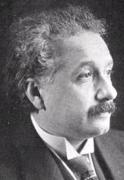Clueless?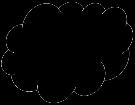What's he talking about?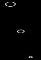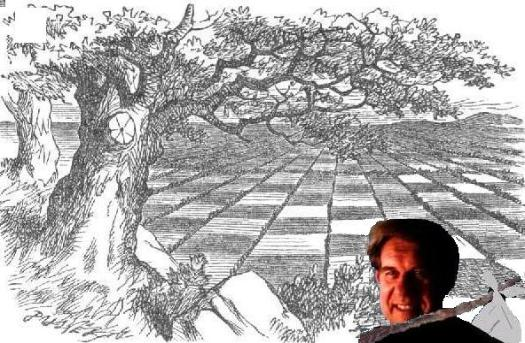Fig. 1   Al’s parallel bars
 According to the relativistic definition lines d, e and f are also parallel. But who in the world would recognize these lines as parallel other than a relativistIn relativity, line c is parallel to lines a and b because it doesn’t touch either one whereas lines a and b are not paralle to each other because they have one point (P)in common. Hence, in relativity, 2 lines that are parallel to a third may not be parallelto each other. However, by simply removing the solid that serves as a backdrop and which is not provided for in the definition of parallel, I show that lines a and c meet if extended far enough!Adapted for the Internet from:   Why God Doesn't Exist
 A mathematician proves the definition of parallel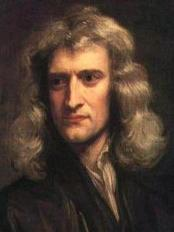So Al?   How far should I walk beforeI certify that they run parallel?

Pages in this module:

2.   This page:   A mathematician proves the definition of parallel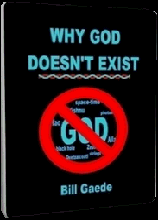________________________________________________________________________________________